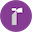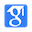# Explaining and Harnessing Adversarial Examplesinternational conference on learning representations, 2014.

EI
Keywords:
art neural networkexamples03 aprilspeculative explanationspeculative hypothesisadversarial exampleMore(12+)
Weibo:
To explain why mutiple classifiers assign the same class to adversarial examples, they hypothesize that neural networks trained with current methodologies all resemble the linear classifier learned on the same training set

Abstract:

Several machine learning models, including neural networks, consistently misclassify adversarial examples---inputs formed by applying small but intentionally worst-case perturbations to examples from the dataset, such that the perturbed input results in the model outputting an incorrect answer with high confidence. Early attempts at exp...More

Code:

Data:

Introduction
• Szegedy et al  made an intriguing discovery: several machine learning models, including state-of-the-art neural networks, are vulnerable to adversarial examples.
• Their explanation suggests a fundamental tension between designing models that are easy to train due to their linearity and designing models that use nonlinear effects to resist adversarial perturbation.
• This explanation shows that a simple linear model can have adversarial examples if its input has sufficient dimensionality.
Highlights
• Szegedy et al  made an intriguing discovery: several machine learning models, including state-of-the-art neural networks, are vulnerable to adversarial examples
• Ian Goodfellow, Jonathon Shlens and ChristiaEnXSPzLeAgIeNdIyNGICALRND20H15AR(INCELSRSI2N0G15A) DVERSARIAL EXAMPLES03 April 2018 4 / 18. Their explanation suggests a fundamental tension between designing models that are easy to train due to their linearity and designing models that use nonlinear effects to resist adversarial perturbation
• We can make many infinitesimal changes to the input that add up to one large change to the output. This explanation shows that a simple linear model can have adversarial examples if its input has sufficient dimensionality
• Recall that without adversarial training, this same kind of model had an error rate of 89.4% on adversarial examples based on the fast gradient sign method
• An intriguing aspect of adversarial examples is that an example generated for one model is often misclassified by other models, even when they have different architecures or were trained on disjoint training sets
• To explain why mutiple classifiers assign the same class to adversarial examples, they hypothesize that neural networks trained with current methodologies all resemble the linear classifier learned on the same training set
Results
• Previous explanations for adversarial examples invoked hypothesized properties of neural networks, such as their supposed highly non-linear nature.
• They hypothesize that neural networks are too linear to resist linear adversarial perturbation.
• This linear behavior suggests that cheap, analytical perturbations of a linear model should damage neural networks.
• Let θ be the parameters of a model, x the input to the model, y the targets associated with x and J(θ, x, y ) be the cost used to train the neural network.
• They find that using = .25, the authors cause a shallow softmax classifier to have an error rate of 99.9% with an average confidence of 79.3% on the MNIST test set.
• A maxout network misclassifies 89.4% of the adversarial examples with an average confidence of 97.6%.
• The criticism of deep networks as vulnerable to adversarial examples is somewhat misguided, because unlike shallow linear models, deep networks are at least able to represent functions that resist adversarial perturbation.
• The authors found that training with an adversarial objective function based on the fast gradient sign method was an effective regularizer: J(θ, x, y ) = αJ(θ, x, y ) + (1 − α)J(θ, x + sign(∇x J(θ, x, y ))
• Five different training runs result in four trials that each had an error rate of 0.77% on the test set and one trial that had an error rate of 0.83%.
• Recall that without adversarial training, this same kind of model had an error rate of 89.4% on adversarial examples based on the fast gradient sign method.
• When the adversarially trained model does misclassify anadversarial example, its predictions are still highly confident.
Conclusion
• An intriguing aspect of adversarial examples is that an example generated for one model is often misclassified by other models, even when they have different architecures or were trained on disjoint training sets.
• To explain why mutiple classifiers assign the same class to adversarial examples, they hypothesize that neural networks trained with current methodologies all resemble the linear classifier learned on the same training set.
• They generated adversarial examples on a deep maxout network and classified these examples using a shallow softmax network and a shallow RBF network.
Summary
• Szegedy et al  made an intriguing discovery: several machine learning models, including state-of-the-art neural networks, are vulnerable to adversarial examples.
• Their explanation suggests a fundamental tension between designing models that are easy to train due to their linearity and designing models that use nonlinear effects to resist adversarial perturbation.
• This explanation shows that a simple linear model can have adversarial examples if its input has sufficient dimensionality.
• Previous explanations for adversarial examples invoked hypothesized properties of neural networks, such as their supposed highly non-linear nature.
• They hypothesize that neural networks are too linear to resist linear adversarial perturbation.
• This linear behavior suggests that cheap, analytical perturbations of a linear model should damage neural networks.
• Let θ be the parameters of a model, x the input to the model, y the targets associated with x and J(θ, x, y ) be the cost used to train the neural network.
• They find that using = .25, the authors cause a shallow softmax classifier to have an error rate of 99.9% with an average confidence of 79.3% on the MNIST test set.
• A maxout network misclassifies 89.4% of the adversarial examples with an average confidence of 97.6%.
• The criticism of deep networks as vulnerable to adversarial examples is somewhat misguided, because unlike shallow linear models, deep networks are at least able to represent functions that resist adversarial perturbation.
• The authors found that training with an adversarial objective function based on the fast gradient sign method was an effective regularizer: J(θ, x, y ) = αJ(θ, x, y ) + (1 − α)J(θ, x + sign(∇x J(θ, x, y ))
• Five different training runs result in four trials that each had an error rate of 0.77% on the test set and one trial that had an error rate of 0.83%.
• Recall that without adversarial training, this same kind of model had an error rate of 89.4% on adversarial examples based on the fast gradient sign method.
• When the adversarially trained model does misclassify anadversarial example, its predictions are still highly confident.
• An intriguing aspect of adversarial examples is that an example generated for one model is often misclassified by other models, even when they have different architecures or were trained on disjoint training sets.
• To explain why mutiple classifiers assign the same class to adversarial examples, they hypothesize that neural networks trained with current methodologies all resemble the linear classifier learned on the same training set.
• They generated adversarial examples on a deep maxout network and classified these examples using a shallow softmax network and a shallow RBF network.
Reference
• C. Szegedy, W. Zaremba, I. Sutskever, J. Bruna, D. Erhan, I. Goodfellow, and R. Fergus. Intriguing properties of neural networks. arXiv preprint arXiv:1312.6199, 2013.• Ian Goodfellow, Jonathon Shlens and ChristiaEnXSPzLeAgIeNdIyNGICALRND20H15AR(INCELSRSI2N0G15A) DVERSARIAL EXAMPLE0S3 April 2018 18 / 18